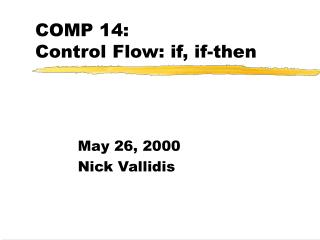DownloadDownload PresentationCOMP 14: Control Flow: if, if-then

# COMP 14: Control Flow: if, if-then

Télécharger la présentation## COMP 14: Control Flow: if, if-then

- - - - - - - - - - - - - - - - - - - - - - - - - - - E N D - - - - - - - - - - - - - - - - - - - - - - - - - - -
##### Presentation Transcript

1. COMP 14: Control Flow: if, if-then May 26, 2000 Nick Vallidis

2. Review • We talked about input and output • What symbols do we use to force the order of operations? • What are the equality operators? • What are the relational operators? • What are the logical operators?

3. Let's try this again • There are class methods and what I'll call object methods. • Class methods are called using the name of the class: • Object methods are called using an instantiated object: result = Math.abs(-17.2); String greeting = new String("Hello, "); greeting = greeting.concat("everyone");

4. What's the difference? • The difference is that class methods have static in front of them when they are defined

5. Today • Control Flow • if statement • if-else statement • nested if and if-else statements

6. Back to the cake • To bake a cake we need: • ingredients • recipe • Similarly for a program we need: • data • algorithm

7. Yep, time for the algorithm • We've already talked some about representing algorithms: • assignment • expressions • methods • But, we need to be able to control the flow of the program...

8. Control Flow • Generally, Java statements are just executed in order, one after another • This is pretty dull. We couldn't even make a calculator… • It would be nice to be able to control which statements get executed (we need to make decisions!) p. 110

9. Good news! • Two types of statements that modify flow: • conditional statements • repetition statements (loops) • Conditional Statements • if, if-else, switch • Repetition Statements • while, do, for p. 110

10. if, if-else • Today, we'll cover these two • We'll come back to switch at a later date • We'll talk about loops next week

11. if • Here is the general form for an if statement: if (condition) statement; p. 111

12. if • Here is the general form for an if statement: if is a Java reserved word if (condition) statement;

13. if • Here is the general form for an if statement: condition is a boolean expression It must evaluate to true or false if is a Java reserved word if (condition) statement;

14. if • Here is the general form for an if statement: condition is a boolean expression It must evaluate to true or false if is a Java reserved word if (condition) statement; If the condition evaluates to true, then the statement is executed, otherwise it is skipped

15. if • Here is the general form for an if statement: if (condition) statement; This indent is VERY important. The compiler doesn't need it, but it helps people read the program

16. Example int age = 22; if (age < 18) System.out.println("Can't vote!"); System.out.println("Always printed");

17. condition evaluated true false statement if Control Flow Diagram if (condition) statement;

18. Another example int year = 2000; if (year == 2000) System.out.println("Sydney Olympics!"); System.out.println("Always printed");

19. Another example int age = 12; if ((age <=12) || (age >= 65)) System.out.println("Discount!"); System.out.println("Always printed");

20. if-else • Here is the general form for an if-else statement: if (condition) statement1; else statement2; p. 114

21. if-else • If the condition is true then statement1 is executed. If the condition is false then statement2 is executed. Never both! if (condition) statement1; else statement2;

22. if-else Diagram condition evaluated if (condition) statement1; else statement2; true false statement2 statement1

23. if-else example int age = 22; if (age < 18) System.out.println("Can't vote!"); else System.out.println("Can vote!"); System.out.println("Always printed");

24. Block Statements • What if we need to do use more than one statement in an if or if-else? • You can use the braces ({ and }) to make a block statement p. 115

25. Example int age = 22; boolean canVote = true; if (age < 18) { System.out.println("Can't vote!"); canVote = false; } System.out.println("Always printed");

26. General format { statement1; statement2; . . . statementn; }

27. Recommendation • A block statement doesn't have to contain more than 1 statement • I recommend always using the block statement form with if and if-else: if (age < 18) { System.out.println("Can't vote!"); }

28. Nested if and if-else • The statements you execute could be another if or if-else!

29. Nested if and if-else if (age > 12) { if (age > 65) System.out.println("Discount"); else System.out.println("Normal price"); } else System.out.println("Child Discount");

30. Examples

31. Homework • Read 3.1-3.2, 3.4 again • Assignment P2 goes out today and is due on Thursday